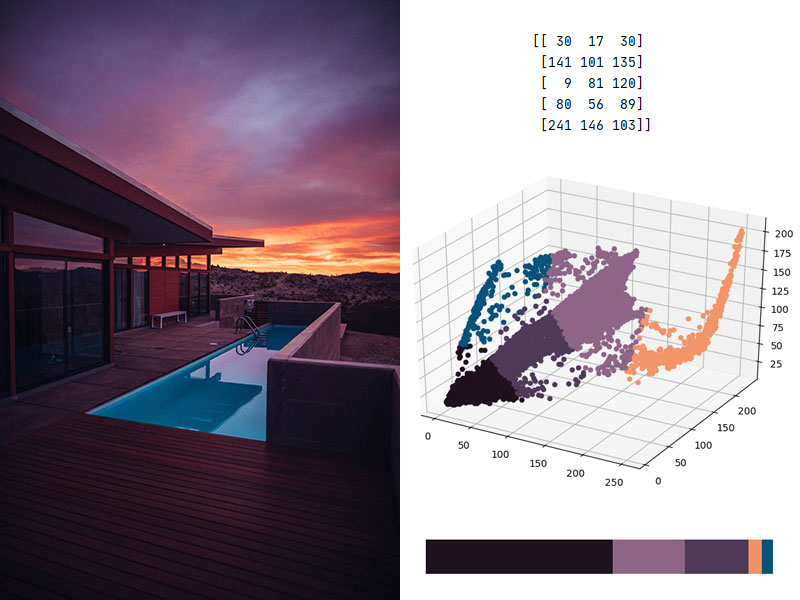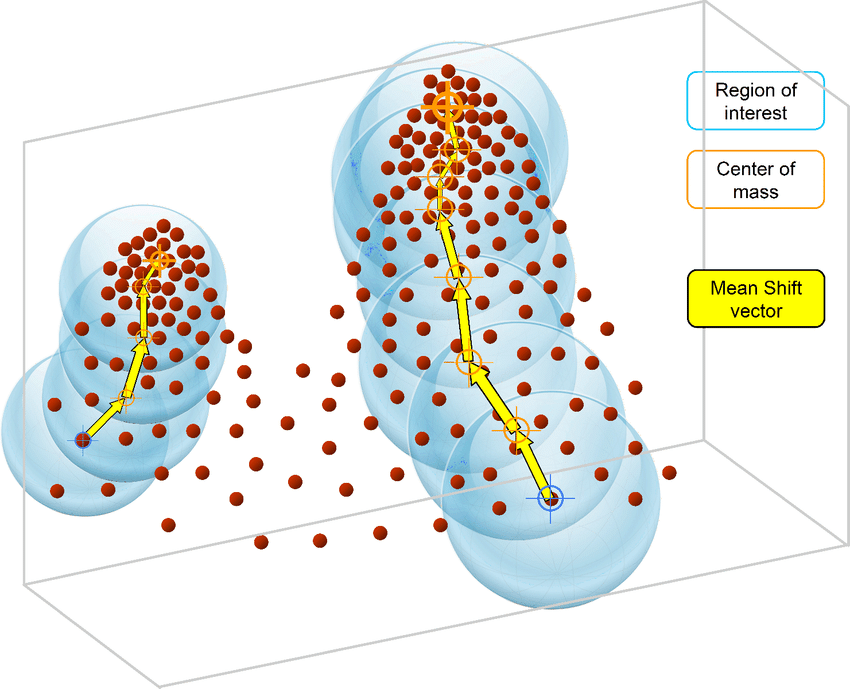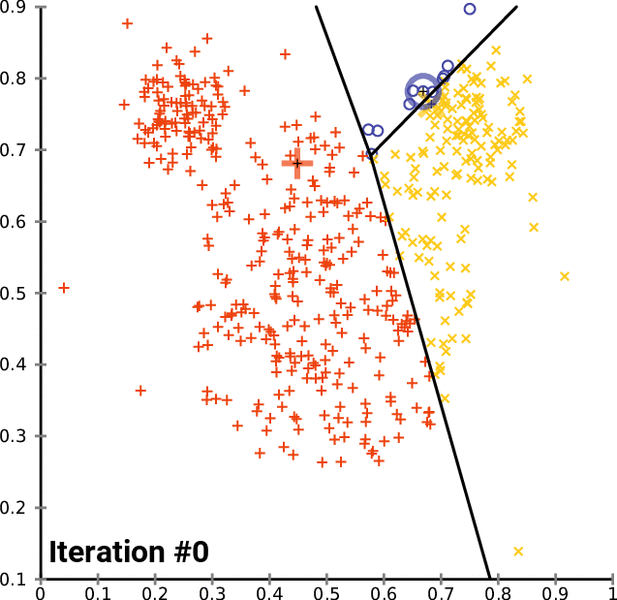## Finding the dominant colors in an image with k-means

Working with images can be a very time-consuming task, especially if you have many images to work on. Machine learning can thus be a great time-saver for various image analysis and editing tasks, such as finding the dominant colors of an image thanks to the K-means clustering algorithm.## How to program the Mean Shift algorithm

Mean Shift is an unsupervised machine learning algorithm. It is a hierarchical data clustering algorithm that finds the number of clusters a feature space should be divided into, as well as the location of the clusters and their centers. It works by grouping data points according to a “bandwidth”, a distance around data points, and converging the clusters’ centers towards the densest regions of data.Continue reading “How to program the Mean Shift algorithm”

## How to program the K-Means clustering algorithm

K-Means is a popular unsupervised machine learning algorithm for data clustering. A typical start for flat clustering, the K-Means algorithm works by defining a number K of clusters to be extracted by the algorithm. With this K number given, the algorithm will then find the best “centroids” to cluster the data around.Continue reading “How to program the K-Means clustering algorithm”

## Conceptual and mathematical summary for machine learning

Machine learning makes use of multiple mathematical formulas and relations to implement the different tasks it can handle. Gathered in the following “cheat sheets” by Afshine and Shervine Amidi, the concepts for supervised and unsupervised learning, deep learning together with machine learning tips and tricks, probabilities, statistics algebra and calculus reminders, are all presented in details with the underlying math.Based on the Stanford course on Machine Learning (CS 229), the cheat sheets summarize the important concepts of each branch with simple explanations and diagrams, such as the following table cover underfitting and overfitting.

 Underfitting Just right Overfitting Symptoms • High training error • Training error close to test error • High bias • Training error slightly lower than test error • Very low training error • Training error much lower than test error • High variance Regression illustrationClassification illustrationDeep learning illustrationPossible remedies • Complexify model • Add more features • Train longer • Perform regularization • Get more data

The main machine learning cheat sheets can be found here:

Other mathematics and coding cheat sheets can be found here:

The complete cheat sheets can also be found on Github.